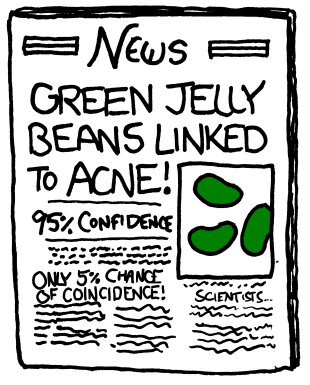Posted by Ian Feller on 3/25/15 1:29 PMFind me on:

--------------------------------------------------------

In February 2015, Basic and Applied Social Psychology (BASP) announced a ban on the use of the null hypothesis significance testing procedure and confidence intervals from future publications in its journal.1 This appears to be a very drastic move on BASP’s part. Why ban the use of hypothesis testing, which is often an integral component of research and statistics? Although BASP’s ban appears radical, the misapplication of null hypothesis testing can lead to inaccurate conclusions that others believe as truth and act on.

## What is Hypothesis Testing?

A null hypothesis significance test is a method of determining if there is a ‘significant’ difference between two groups. For example, we may want to know if the fuel efficiency of premium gasoline is statistically different from that of regular gasoline. A typical approach by researchers is to calculate the average fuel efficiency of a sample of cars that use premium gasoline, and compare it to the average fuel efficiency of a sample of cars that use regular gasoline.

To test whether the difference between gasolines is ‘significant,’ we compute and interpret a p-value, which measures the risk that results are incorrect (that is, different from zero purely by chance when the actual coefficient is zero). To interpret the p-value, researchers compare the computed value to a threshold, which is also known as the significance level. Typically, the threshold is held at 5%, but can be set at 1% or 10%.

So, how do we link the p-value to our research question, "Is there a significant difference in fuel efficiency due to gasoline type?" We answer our question by testing a null-hypothesis of the question (i.e. there is no difference in fuel efficiency). If the p-value is less than the threshold, we ‘reject’ the null hypothesis, rejecting the notion that there is no difference in fuel efficiency among gasoline types, and conclude there is a significant difference between the fuel efficiency of gasoline types. However, if the p-value is greater than the threshold, we ‘fail to reject’ the null hypothesis. This means that we are unable to conclude if regular and premium gas provide similar or different levels of fuel efficiency.

## What is the controversy?

Although hypothesis testing is widely used by researchers, there are limits to the technique. Controversy arises when a p-value is misinterpreted as a measure of the accuracy of the results of the study. This can lead to publication bias, in which journals are more likely to publish papers with statistically significant results. This may encourage scientists to design experiments and explore relationships in which p-values are low to begin with. Furthermore, as data become more accessible and widely distributed, it has become easier to re-run tests using different data or slightly different variables until the desired result is produced. As British economist Ronald Coase cautions, "If you torture the data long enough, it will confess to anything."

In our gasoline example, let’s set our threshold at 5% and assume there is a significant difference in fuel efficiency between regular and premium gasoline. If the calculated p-value is less than the 5% threshold, we ‘reject’ the null hypothesis. Therefore, we can be 95% confident that our results are representative of the population. If we perform this experiment 20 times, 19 of the results would be correct. 1 in 20 times, however, we would believe there is no difference in gasoline efficiencies when a difference actually exists. This is an example of a ‘false negative,’ a result that appears negative when it should not. The reverse can also be true. A result can appear positive when in actuality is should not, as depicted in the following cartoon.95% confidence implies 1 in every 20 cases will produce a false positive. As a greater number of tests are performed, the amount of false positives will increase. In this facetious case, scientists conclude green jelly beans are linked to acne. Source:  http://xkcd.com/882/

## How does this relate to Summit?

As a leading analytics firm, Summit is keenly interested in new developments in statistics to provide the highest quality solutions to our clients. Although we frequently use hypothesis tests to inform our analyses, we do not rely on this technique as the sole measure of soundness.

It is important to avoid the following common errors when evaluating the results of a hypothesis test.

1. Statistically significant findings (with p-values less than 5%) are assumed to result from real treatment effects. However, by definition 1 in 20 tests will provide a false positive.
2. Studies with small sample sizes and p-values greater than 5% are interpreted as “not significant” and ignored. However, studies with small sample sizes are prone to produce results that are not statistically significant, but may have important clinical or practical implications.
3. Studies with large sample sizes and statistically significant findings are not always important. Small differences may be statistically different, but the implications of the finding may have limited to no practical application.

In short, hypothesis testing provides guidance on whether observations could have occurred by chance, and researchers should not use p-values alone to determine the quality or accuracy of a study. In our fuel efficiency example, researchers should apply hypothesis testing to measure the risk of drawing a wrong conclusion. Furthermore, they should interpret p-values in relation to the sample size and practical application of findings.

This post was written with the help of Doja Khandkar. Dr. Ed Dieterle and Dr. George Cave provided editorial and technical guidance.Topics: data analytics